The big clock

The big clock hands stopped at a random moment. What is the probability that:

a) a small hand showed the time between 1:00 and 3:00?
b) the big hand was in the same area as a small hand in the role of a)?
c) did the hours just show the time between 21:00 and 22:30?

Result

p1 =  25 %
p2 =  25 %
p3 =  12.5 %

Solution:Leave us a comment of example and its solution (i.e. if it is still somewhat unclear...):Be the first to comment!To solve this example are needed these knowledge from mathematics:

Would you like to compute count of combinations?

Next similar examples:

1. First manWhat is the likelihood of a random event where are five men and seven women first will leave the man?
2. Today in schoolThere are 9 girls and 11 boys in the class today. What is the probability that Suzan will go to the board today?
3. Seagull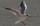Artificially created island in the shape of a circle with a radius of 50 m is overgrown with grass. The only exception is a landing area for helicopters in the shape of a rectangle measuring 15 m and 8 m. What is the probability that the flying seagull (wi
4. Components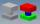In the box are 8 white, 4 blue and 2 red components. What is the probability that we pull one white, one blue and one red component without returning?
5. The diceWhat is the probability of events that if we throw a dice is rolled less than 6?
6. Sum 10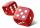What is the probability that two dice throw twice in a row will result the sum of 10?
7. Probability - tickets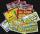What is the probability that when you have 25 tickets in 5000 that you wins the first (one) prize?
8. In the orchardIn the orchard, they planted 25 apple trees, 20 pears, 15 plums and 40 marbles. A strong late frost, however, destroyed a fifth of all new trees. Unfortunately, it was all the trees of one kind of fruit. What is the probability that the plums have died out
9. Probability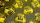What are the chances that the lottery, in which the numbers are drawn 5 of 50 you win the first prize?
10. Theorem proveWe want to prove the sentence: If the natural number n is divisible by six, then n is divisible by three. From what assumption we started?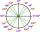What is greater angle? -0.7 radians or -0.7π radians?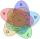If probabilities of A, B and A ∩ B are P (A) = 0.62 P (B) = 0.78 and P (A ∩ B) = 0.26 calculate the following probability (of union. intersect and opposite and its combinations):A certain species of tree grows an average of 0.5 cm per week. Write an equation for the sequence that represents the weekly height of this tree in centimeters if the measurements begin when the tree is 200 centimeters tall.Find the reference angle of each angle: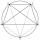How to divide the circle into 10 parts (geometrically)?Solve equations (use adding and subtracting of linear equations): -4x+11y=5 6x-11y=-5Find the nineteenth member of the arithmetic sequence: a1=33 d=5 find a19